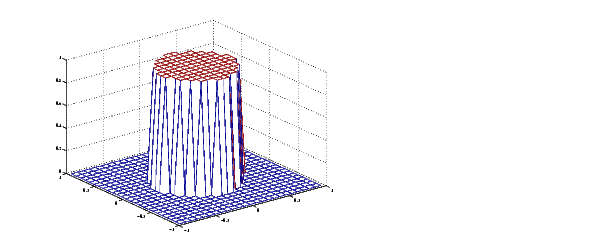Image Processing Toolbox User's GuideCreating the Desired Frequency Response Matrix

The filter design functions `fsamp2`, `fwind2`, and `fwind2` all create filters based on a desired frequency response magnitude matrix. Frequency response is a mathematical function describing the gain of a filter in response to different input frequencies.

You can create an appropriate desired frequency response matrix using the `freqspace` function. `freqspace` returns correct, evenly spaced frequency values for any size response. If you create a desired frequency response matrix using frequency points other than those returned by `freqspace`, you might get unexpected results, such as nonlinear phase.

For example, to create a circular ideal lowpass frequency response with cutoff at 0.5, use

• ```[f1,f2] = freqspace(25,'meshgrid');
Hd = zeros(25,25); d = sqrt(f1.^2 + f2.^2) < 0.5;
Hd(d) = 1;
mesh(f1,f2,Hd)```

Ideal Circular Lowpass Frequency Response

Note that for this frequency response, the filters produced by `fsamp2`, `fwind1`, and `fwind2` are real. This result is desirable for most image processing applications. To achieve this in general, the desired frequency response should be symmetric about the frequency origin (`f1` `=` `0`, `f2` `=` `0`).Windowing Method Computing the Frequency Response of a Filter